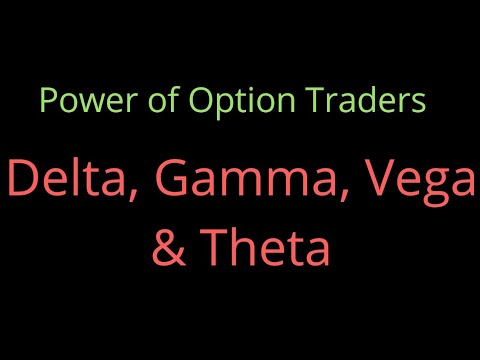## Trending

recent

For an options trader to be successful in the market there are several forces which need to work in the option traders' favour. These forces are collectively called ‘The Option Greeks’.
These forces influence an option contract in real-time, affecting the premium to either increase or decrease on a minute by minute basis.
To make matters complicated, these forces not only influence the premiums directly but also influence each other.

These forces are:

Delta – Measures the rate of change of options premium based on the directional movement of the underlying

Gamma – Rate of change of delta itself

Vega – Rate of change of premium based on the change in volatility

Theta – Measures the impact on premium based on time left for expiry.

Delta
The Delta measures how the value of an option changes for the change in the underlying.

In simpler terms, the Delta of an option helps us answer questions of this sort – By how many points will the option premium change for every 1 point change in the underlying?

When the value of the spot changes, so does the option premium.
Precisely – the call option premium increases with the increase in the spot value and vice versa.
The Option Greek’s ‘Delta’ captures the effect of the directional movement of the market on the Option’s premium.
The delta is a number which varies –

Between 0 and 1 for a call option
Between -1 and 0 for a put option.
Delta depicts the probability of Option expiring In the Money
Higher the Delta (positive/negative) higher the probability of Option expiring In the Money
Lower the Delta (positive /negative) lower the probability of Option expiring In the Money
Call options has a +ve delta. A Call option with a delta of 0.5 indicates that for every 1 point gain/loss in the underlying the call option premium gains/losses 0.5 points
Put options has a –ve delta. A Put option with a delta of -0.5 indicates that for every 1 point loss/gain in the underlying the put option premium gains/losses 0.5 points
OTM options have a delta value between 0 and 0.5, ATM option has a delta of 0.5, and the ITM option has a delta between 0.5 and 1.

Look at the X-axis – starting from left the moneyness increases as the spot price traverses from OTM to ATM to ITM
Look at the delta line (blue line) – as and when the spot price increases so does the delta
Notice at OTM the delta is flattish near 0 – this also means irrespective of how much the spot price falls ( going from OTM to deep OTM) the option’s delta will remain at 0
Remember the call option’s delta is lower bound by 0
When the spot moves from OTM to ATM the delta also starts to pick up (remember the option’s moneyness also increases)
Notice how the delta of option lies within 0 to 0.5 range for options that are less than ATM
At ATM, the delta hits a value of 0.5

Ø When the spot moves along from the ATM towards ITM the delta starts to move beyond the 0.5 mark
Ø Notice the delta starts to flatten out when it hits a value of 1
Ø This also implies that as and when the delta moves beyond ITM to say deep ITM the delta value does not change. It stays at its maximum value of 1.

Value of Delta

Properties of Delta
Ø The delta is additive in nature
Ø The delta of a futures contract is always 1
Ø Two ATM option is equivalent to owning a future contract
Ø Positions such as this – which have a combined delta of 0 are also called ‘Delta Neutral’ positions
Ø However Delta neutral positions react to other variables like Volatility and Time.

Gamma

Ø GAMMA is the rate of change of delta for change in underlying
Ø GAMMA is Positive for “LONG OPTION" and negative for "SHORT OPTION"
Ø Gamma is maximum for ATM option and decreases as the option moves from ITM to OTM
Ø Gamma accelerates the momentum in premium

Ø In low volatility scenario, Gamma is highest for ATM option and decreases for ITM and OTM option
Ø In High volatility scenario, Gamma tends to be stable for all ITM, ATM and OTM option as time value is high for all three option and no major changes happen as the option moves from ITM, OTM to ATM
Ø As time to expiry comes closer, Gamma becomes sensitive to ATM option compared to ITM, OTM
Ø For a long option, high Gamma is good if our forecast is correct.
Ø For Short option, high Gamma is worst if your forecast is incorrect as an option will work against you at an accelerated pace.

Theta
Ø Theta refers to how much an option will lose over time. It is either expressed as Total theta or Theta per day.
Ø Long Option has Negative Theta while Short Option has Positive Theta.

Ø Option sellers are always compensated for the time risk
Ø Premium = Intrinsic Value + Time Value
Ø All else equal, options lose money daily owing to Theta
Ø Time moves in a single direction hence Theta is a positive number
Ø Theta is a friendly Greek to option sellers
Ø When you short naked options at the start of the series you can pocket a large time value but the fall in premium owing to time is low
Ø When you short option close to expiry the premium is low (thanks to time value) but the fall in premium is rapid.

Vega:

Ø Vega is positive for Long Options and negative on Short Option
Ø Vega is maximum when Time to expiry is highest
Ø As expiry comes closer Vega becomes sensitive for ATM option
Ø As Implied Volatility of the options increase, Vega of all option is similar to that of

What are option Greeks and how to interpret them during trading?Reviewed by Kumar Chandan on February 02, 2020 Rating: 5